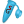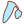Promoterless adenoviral Gateway® destination vector for inserting a gene expression cassette.

Sequence Author: Thermo Fisher (Invitrogen)

Explore Over 2.7k Plasmids: Gateway® Cloning Vectors | More Plasmid Sets
No matches
 BstBI  (29,683)1 site T T C G A A A A G C T T
 SpeI  (25,803)1 site A C T A G T T G A T C A
 MreI  (25,110)1 site C G C C G G C G G C G G C C G C
 AsiSI  (20,163)1 site G C G A T C G C C G C T A G C G
 PmeI  (11,979)1 site G T T T A A A C C A A A T T T G
 adenoviral DNA2234 .. 32,782  =  30,549 bpsequences from human adenovirus serotype 5, lacking the E1 and E3 regions
 adenoviral DNA2234 .. 32,782  =  30,549 bpsequences from human adenovirus serotype 5, lacking the E1 and E3 regions
 AmpR33,746 .. 34,606  =  861 bp286 amino acids  =  ~31.6 kDa2 segments   Segment 2:     33,746 .. 34,537  =  792 bp   263 amino acids  =  ~29.0 kDaProduct: β-lactamaseconfers resistance to ampicillin, carbenicillin, and related antibiotics
 AmpR33,746 .. 34,606  =  861 bp286 amino acids  =  ~31.6 kDa2 segments   Segment 1:  signal sequence     34,538 .. 34,606  =  69 bp   23 amino acids  =  2.6 kDaProduct: β-lactamaseconfers resistance to ampicillin, carbenicillin, and related antibiotics
 AmpR33,746 .. 34,606  =  861 bp286 amino acids  =  ~31.6 kDa2 segmentsProduct: β-lactamaseconfers resistance to ampicillin, carbenicillin, and related antibiotics
 CmR745 .. 1404  =  660 bp219 amino acids  =  25.7 kDaProduct: chloramphenicol acetyltransferaseconfers resistance to chloramphenicol
 CmR745 .. 1404  =  660 bp219 amino acids  =  25.7 kDaProduct: chloramphenicol acetyltransferaseconfers resistance to chloramphenicol
 ori33,006 .. 33,594  =  589 bphigh-copy-number ColE1/pMB1/pBR322/pUC origin of replication
 ori33,006 .. 33,594  =  589 bphigh-copy-number ColE1/pMB1/pBR322/pUC origin of replication
 ccdB1746 .. 2051  =  306 bp101 amino acids  =  11.7 kDaProduct: CcdB, a bacterial toxin that poisons DNA gyrasePlasmids containing the ccdB gene cannot be propagated in standard E. coli strains.
 ccdB1746 .. 2051  =  306 bp101 amino acids  =  11.7 kDaProduct: CcdB, a bacterial toxin that poisons DNA gyrasePlasmids containing the ccdB gene cannot be propagated in standard E. coli strains.
 Ad5 Ψ191 .. 341  =  151 bppackaging signal for adenovirus serotype 5
 Ad5 Ψ191 .. 341  =  151 bppackaging signal for adenovirus serotype 5
 attR1512 .. 636  =  125 bprecombination site for the Gateway® LR reaction
 attR1512 .. 636  =  125 bprecombination site for the Gateway® LR reaction
 attR22092 .. 2216  =  125 bprecombination site for the Gateway® LR reaction
 attR22092 .. 2216  =  125 bprecombination site for the Gateway® LR reaction
 AmpR promoter34,607 .. 34,711  =  105 bp
 AmpR promoter34,607 .. 34,711  =  105 bp
 5' ITR1 .. 103  =  103 bpinverted terminal repeat of human adenovirus serotype 5
 5' ITR1 .. 103  =  103 bpinverted terminal repeat of human adenovirus serotype 5
 lac UV5 promoter661 .. 691  =  31 bp3 segments   Segment 1:  -35     661 .. 666  =  6 bpE. coli lac promoter with an "up" mutation
 lac UV5 promoter661 .. 691  =  31 bp3 segments   Segment 2:     667 .. 684  =  18 bpE. coli lac promoter with an "up" mutation
 lac UV5 promoter661 .. 691  =  31 bp3 segments   Segment 3:  -10     685 .. 691  =  7 bpE. coli lac promoter with an "up" mutation
 lac UV5 promoter661 .. 691  =  31 bp3 segmentsE. coli lac promoter with an "up" mutation
 3' ITR32,680 .. 32,782  =  103 bpinverted terminal repeat of human adenovirus serotype 5
 3' ITR32,680 .. 32,782  =  103 bpinverted terminal repeat of human adenovirus serotype 5
 ORF:  745 .. 1404  =  660 bpORF:  219 amino acids  =  25.7 kDa
 ORF:  5011 .. 5331  =  321 bpORF:  106 amino acids  =  11.7 kDa
 ORF:  6031 .. 6282  =  252 bpORF:  83 amino acids  =  9.4 kDa
 ORF:  7216 .. 7452  =  237 bpORF:  78 amino acids  =  7.9 kDa
 ORF:  12,877 .. 14,592  =  1716 bpORF:  571 amino acids  =  63.3 kDa
 ORF:  14,599 .. 15,195  =  597 bpORF:  198 amino acids  =  22.0 kDa
 ORF:  15,232 .. 16,371  =  1140 bpORF:  379 amino acids  =  42.6 kDa
 ORF:  16,399 .. 16,641  =  243 bpORF:  80 amino acids  =  8.8 kDa
 ORF:  17,563 .. 20,421  =  2859 bpORF:  952 amino acids  =  108.0 kDa
 ORF:  22,456 .. 22,812  =  357 bpORF:  118 amino acids  =  12.8 kDa
 ORF:  23,155 .. 23,541  =  387 bpORF:  128 amino acids  =  14.3 kDa
 ORF:  24,718 .. 25,506  =  789 bpORF:  262 amino acids  =  28.9 kDa
 ORF:  29,578 .. 29,976  =  399 bpORF:  132 amino acids  =  15.0 kDa
 ORF:  30,799 .. 31,296  =  498 bpORF:  165 amino acids  =  18.7 kDa
 ORF:  32,851 .. 33,087  =  237 bpORF:  78 amino acids  =  8.9 kDa
 ORF:  2330 .. 2752  =  423 bpORF:  140 amino acids  =  14.5 kDa
 ORF:  9152 .. 9565  =  414 bpORF:  137 amino acids  =  14.4 kDa
 ORF:  11,039 .. 12,796  =  1758 bpORF:  585 amino acids  =  65.3 kDa
 ORF:  16,007 .. 16,486  =  480 bpORF:  159 amino acids  =  17.4 kDa
 ORF:  16,724 .. 17,476  =  753 bpORF:  250 amino acids  =  27.0 kDa
 ORF:  25,895 .. 26,578  =  684 bpORF:  227 amino acids  =  24.7 kDa
 ORF:  26,579 .. 26,902  =  324 bpORF:  107 amino acids  =  12.3 kDa
 ORF:  27,320 .. 27,682  =  363 bpORF:  120 amino acids  =  13.7 kDa
 ORF:  31,310 .. 31,648  =  339 bpORF:  112 amino acids  =  12.5 kDa
 ORF:  32,933 .. 33,331  =  399 bpORF:  132 amino acids  =  14.8 kDa
 ORF:  1746 .. 2051  =  306 bpORF:  101 amino acids  =  11.7 kDa
 ORF:  6699 .. 7148  =  450 bpORF:  149 amino acids  =  16.7 kDa
 ORF:  8025 .. 8531  =  507 bpORF:  168 amino acids  =  17.7 kDa
 ORF:  9771 .. 11,018  =  1248 bpORF:  415 amino acids  =  47.1 kDa
 ORF:  20,454 .. 21,068  =  615 bpORF:  204 amino acids  =  23.1 kDa
 ORF:  22,200 .. 25,205  =  3006 bpORF:  1001 amino acids  =  110.0 kDa
 ORF:  27,828 .. 29,630  =  1803 bpORF:  600 amino acids  =  63.6 kDa
 ORF:  33,876 .. 34,142  =  267 bpORF:  88 amino acids  =  ~9.3 kDa
 ORF:  4058 .. 4405  =  348 bpORF:  115 amino acids  =  12.2 kDa
 ORF:  7304 .. 9265  =  1962 bpORF:  653 amino acids  =  74.7 kDa
 ORF:  10,280 .. 10,573  =  294 bpORF:  97 amino acids  =  10.5 kDa
 ORF:  17,186 .. 17,515  =  330 bpORF:  109 amino acids  =  11.4 kDa
 ORF:  21,164 .. 22,753  =  1590 bpORF:  529 amino acids  =  59.1 kDa
 ORF:  25,148 .. 25,438  =  291 bpORF:  96 amino acids  =  10.5 kDa
 ORF:  28,001 .. 28,471  =  471 bpORF:  156 amino acids  =  16.6 kDa
 ORF:  28,805 .. 29,050  =  246 bpORF:  81 amino acids  =  8.7 kDa
 ORF:  31,196 .. 31,546  =  351 bpORF:  116 amino acids  =  13.3 kDa
 ORF:  33,746 .. 34,606  =  861 bpORF:  286 amino acids  =  ~31.6 kDa
 ORF:  2812 .. 4170  =  1359 bpORF:  452 amino acids  =  51.2 kDa
 ORF:  5173 .. 5511  =  339 bpORF:  112 amino acids  =  12.8 kDa
 ORF:  7183 .. 7761  =  579 bpORF:  192 amino acids  =  20.8 kDa
 ORF:  9475 .. 9840  =  366 bpORF:  121 amino acids  =  12.7 kDa
 ORF:  11,035 .. 11,295  =  261 bpORF:  86 amino acids  =  9.0 kDa
 ORF:  13,102 .. 13,887  =  786 bpORF:  261 amino acids  =  28.5 kDa
 ORF:  15,364 .. 15,633  =  270 bpORF:  89 amino acids  =  9.5 kDa
 ORF:  16,882 .. 17,430  =  549 bpORF:  182 amino acids  =  18.9 kDa
 ORF:  30,841 .. 31,185  =  345 bpORF:  114 amino acids  =  13.3 kDa
 ORF:  31,984 .. 32,370  =  387 bpORF:  128 amino acids  =  14.3 kDa
 ORF:  32,740 .. 33,036  =  297 bpORF:  98 amino acids  =  10.8 kDa
 ORF:  3195 .. 3419  =  225 bpORF:  74 amino acids  =  8.3 kDa
 ORF:  3918 .. 7088  =  3171 bpORF:  1056 amino acids  =  120.4 kDa
 ORF:  9981 .. 10,349  =  369 bpORF:  122 amino acids  =  13.6 kDa
 ORF:  15,918 .. 16,169  =  252 bpORF:  83 amino acids  =  8.3 kDa
 ORF:  16,368 .. 16,658  =  291 bpORF:  96 amino acids  =  10.9 kDa
 ORF:  17,595 .. 17,870  =  276 bpORF:  91 amino acids  =  10.2 kDa
 ORF:  30,036 .. 30,920  =  885 bpORF:  294 amino acids  =  34.1 kDaSnapGene

SnapGene is the easiest way to plan, visualize and document your everyday molecular biology procedures

• Fast accurate construct design for all major molecular cloning techniques
• Validate sequenced constructs using powerful alignment tools
• Customize plasmid maps with flexible annotation and visualization controls
• Automatically generate a rich graphical history of every edit and procedureSnapGene Viewer

SnapGene Viewer is free software that allows molecular biologists to create, browse, and share richly annotated sequence files.

• Gain unparalleled visibility of your plasmids, DNA and protein sequences
• Annotate features on your plasmids using the curated feature database
• Store, search, and share your sequences, files and maps

The maps, notes, and annotations in the zip file on this page are copyrighted material. This material may be used without restriction by academic, nonprofit, and governmental entities, except that the source must be cited as ’’www.snapgene.com/resources’’. Commercial entities must contact GSL Biotech LLC for permission and terms of use.

Discover the most user-friendly molecular biology experience.